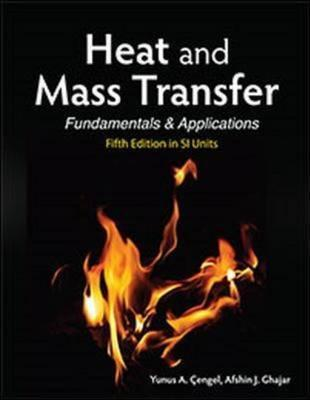•# Heat and Mass Transfer in SI Units Cengel ISBN: 9789814595278

RM 80.00
- +

This text provides a complete coverage of the basic principles of heat transfer and a broad range of applications. Heat and Mass Transfer: Fundamentals and Applications by Yunus Çengel and Afshin Ghajar provide the perfect blend of fundamentals and applications. The text provides a highly intuitive and practical understanding of the material by emphasizing the physics and the underlying physical phenomena involved.

This text covers the standard topics of heat transfer with an emphasis on physics and real-world every day applications, while de-emphasizing the intimidating mathematical aspects. This approach is designed to take advantage of students' intuition, making the learning process easier and more engaging.

Chapter 1 Introduction and Basic Concepts

Chapter 2 Heat Conduction Equation

Chapter 4 Transient Heat Conduction

Chapter 5 Numerical Methods in Heat Conduction

Chapter 6 Fundamentals of Convection

Chapter 7 External Forced Convection

Chapter 8 Internal Forced Convection

Chapter 9 Natural Convection

Chapter 10 Boiling and Condensation

Chapter 11 Heat Exchangers

Chapter 12 Fundamentals of Thermal Radiation